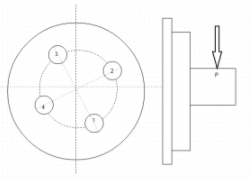Courses

# Test: Eccentric Load On Circular Base

## 5 Questions MCQ Test Machine Design | Test: Eccentric Load On Circular Base

Description
This mock test of Test: Eccentric Load On Circular Base for Mechanical Engineering helps you for every Mechanical Engineering entrance exam. This contains 5 Multiple Choice Questions for Mechanical Engineering Test: Eccentric Load On Circular Base (mcq) to study with solutions a complete question bank. The solved questions answers in this Test: Eccentric Load On Circular Base quiz give you a good mix of easy questions and tough questions. Mechanical Engineering students definitely take this Test: Eccentric Load On Circular Base exercise for a better result in the exam. You can find other Test: Eccentric Load On Circular Base extra questions, long questions & short questions for Mechanical Engineering on EduRev as well by searching above.
QUESTION: 1

### A flange of radius 200mm is fastened to the machine screw by means of four cap screws of pitch circle radius 150mm. The external force P is 20kN which is loaded at 160mm from the machine screw. There are two dowel pins to take shear load. Determine the maximum effective tensile force acting on the bolt. The four bolts are equally spaced radially.

Solution:

Explanation: Maximum force F=2Pl[a+bCos(90/2)] / 4(2a²+b²).

QUESTION: 2

### A flange of radius 200mm is fastened to the machine screw by means of four cap screws of pitch circle radius 150mm. The external force P is 20kN which is loaded at 160mm from the machine screw. There are two dowel pins to take shear load. Determine the core diameter of cap screw if permissible tensile stress in cap screw is 40N/mm².

Solution:

Explanation: Maximum tensile force=4777.6N or πd² x σ/4=4777.6N.

QUESTION: 3

### A round flange bearing is fastened to the machine frame by means of 4 cap screws. The angle made by central axis of bolt 2 and horizontal is θ. Also the radii of flange and pitch circle are a & b respectively. Given: Sum of squares of distance of bolts from axis of tilt is 2a² +b²Q. Are all the four bolts under equal effective tensile load?

Solution:

Explanation: All the bolts are at different distance from the axis of tilt and hence unequally loaded.

QUESTION: 4

Determine the angle θ so that the force in the bolt 1 has maximum value.

Solution:

Explanation:F₁=2Plx(a-b Cos θ)/4(2a²+b²), hence for force to be max Cosθ=-1 or θ=180’.

QUESTION: 5

Which bolt is under maximum stress if dowel pins are used to relieve cap screws from shear stress?

Solution:

Explanation: The screw farthest from the axis of tilt is under maximum stress.